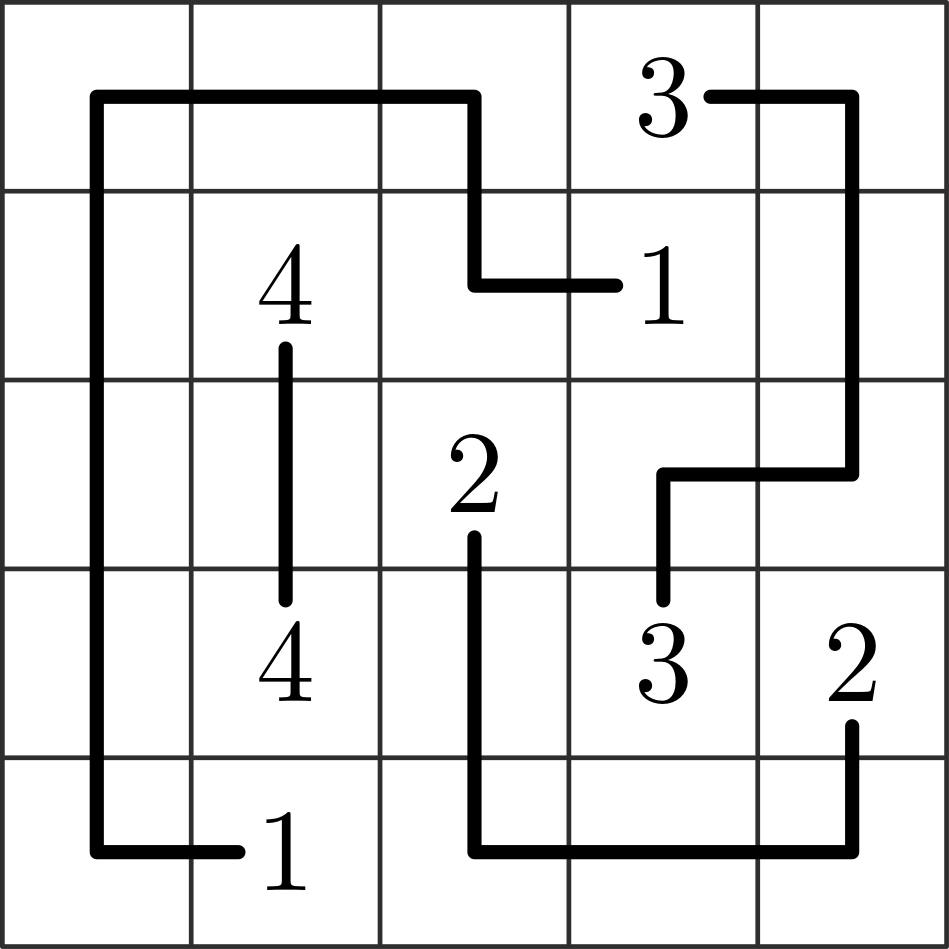A single player game in which the player connects pairs of points in a rectangular grid with paths that traverse all the grid cells and avoid unnecessary turns.## Description

Numberlink is a single player game played on a rectangular grid. Initially, some of the cells of the grid are empty while others contain a number from $1$ to $k$, each of which appears exactly twice.

Each grid cells is adjacent to the cells to its left, right, top, and bottom, if any. The goal is to find a collection of $k$ simple paths (sequences of adjacent cells) such that:

1. The endpoints of the $i$-th path are the two cells containing number $i$;
2. Each cell of the grid belongs to exactly one path; and
3. For each $i=1,\dots,k$ the following holds: once paths $1, \dots, i-1, i+1, k$ are fixed, the $i$-th path performs the minimum number of turns among those that satisfy the previous two conditions.

## Computational complexity

The problem of deciding whether an instance of Numberlink admits a solution is NP-Complete .

The variant in which condition 3 is removed is known as Zig-Zag Numberlink.K. Kotsuma, Y. Takenaga, “NP-Completeness and Enumeration of Number Link Puzzle”, IEICE Technical Report, 2010.

@misc{cog:numberlink,
author = "{CoG contributors}",
title  = "{Numberlink --- Complexity of Games}",
year   = "2022",
}